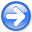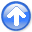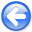Tomographic full waveform inversion and linear modeling of multiple scatteringNext: Gradient computation with FWI Up: Biondi: TFWI and multiple Previous: Introduction

# Conventional Full Waveform Inversion (FWI)

Conventional full waveform inversion is performed by solving the following optimization problem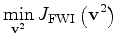(1)

where: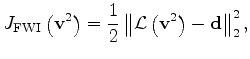(2)is the velocity vector,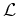is a wave-equation operator non linear with respect to velocity perturbations and the data vector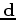is the pressure fieldmeasured at the surface.

The wave-equation operator is evaluated by recursively solving the following finite difference equation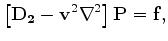(3)

where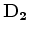is a finite-difference representation of the second derivative in time,is a finite-difference representation of the Laplacian, and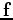is the source function.

SubsectionsTomographic full waveform inversion and linear modeling of multiple scatteringNext: Gradient computation with FWI Up: Biondi: TFWI and multiple Previous: Introduction

2012-10-29